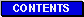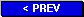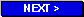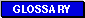Short-Range Actuarial Projections of the Old-Age, Survivors, and Disability Insurance Program, 2001 Actuarial Study No. 115 Chris Motsiopoulos and Tim Zayatz, A.S.A.# Appendix

### A. DETERMINATION OF THE WAGE BASE

The maximum amount of taxable and creditable earnings for Social Security purposes, usually referred to as the wage base, is determined each year as prescribed by law. Currently, the wage base is determined each year based on legislation enacted August 15, 1994.

The wage base for a given year is calculated in the preceding year by multiplying the wage base of \$60,600 for 1994 by the following quotient: the national average wage index for the prior year, divided by the national average wage index for 1992. The result, rounded to the nearest \$300, is the new wage base. The wage base can remain the same or increase. In no case, is the new wage base reduced to an amount below the previous wage base.

The 1994 wage base was \$60,600.
The 1992 national average wage index was \$22,935.42.

Example:

The wage base for calendar year 2001 was determined in 2000 as follows:
$(1994 wage base) x [(1999 average wage index) / (1992 average wage index)]$
$60,6000.00 x [30,469.84 / 22,935.42] = 80,507.46$
Rounding \$80,507.46 to the nearest \$300 results in a wage base for 2001 of \$80,400.

Wage bases for projected years are estimated in the same manner using the projected (or preliminary) average wage index for the year that is 2 years prior to the year for which the wage base is being calculated. Thus, for the 2001 Trustees Report, the 2002 wage base is estimated using the 1994 wage base, the projected 2000 average wage index, and the 1992 average wage index.

List of Studies
December 26, 2001